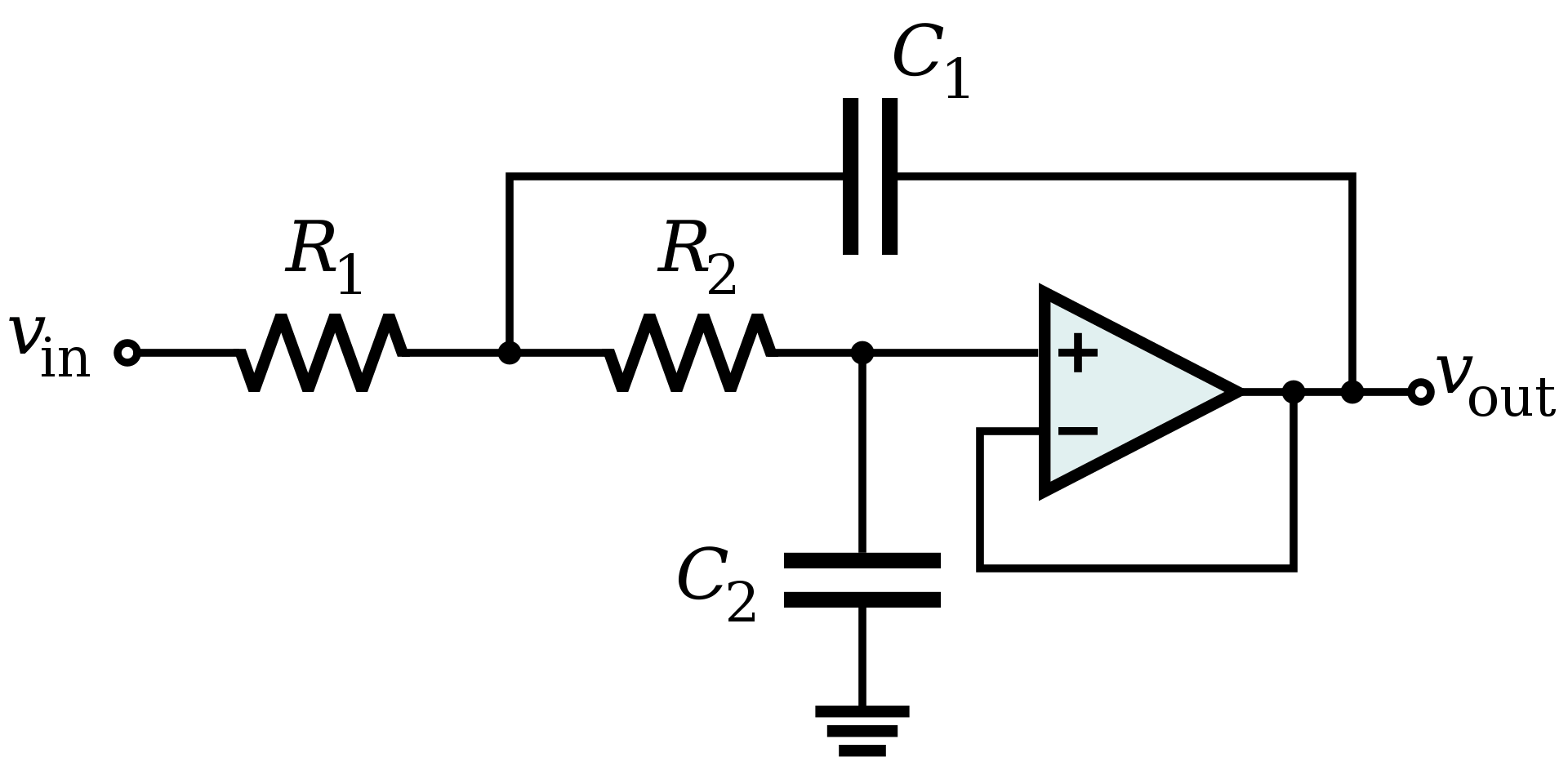# Sallen & Key FilterWritten by

The Sallen & Key circuit is the simplest active 2-pole filter circuit you will find. It uses just two resistors, two capacitors and an op-amp connected as a buffer to realise a low-pass or high-pass filter. It is a simplified form of the VCVS (voltage-controlled voltage source) filter, and was first described in 1955 by R. P. Sallen and E. L Key of MIT.

Its important to note that Sallen & Key is a filter topology – not a filter characteristic such as Butterworth, Bessel, Chebyshev etc. These filter characteristics can be implemented in various filter topologies, including Sallen & Key, by choosing the right component values.

Sallen & Key filters are used mainly in low and high pass configurations, although band-pass is possible with more components. They are very easy to implement and should be your go-to topology if two poles are sufficient for your needs. They can be cascaded, but it’s probably best to use a topology like VCVS if you need more poles.

Figure 1 shows the Sallen & Key implementation for a low-pass filter. A high-pass filter looks exactly the same except the resistors are replaced by capacitors and vice versus. Looking closely, you can see that the circuit is effectively two RC filter sections in series, but with the capacitor of the first stage bootstrapped by the output.Figure 1This is a low-pass implementation of a Sallen & Key filter topology. The high-pass version is identical except the resistors and capacitors are in opposite places. The circuit is effectively a pair of cascaded RC filters with the first stage bootstrapped by the output.

The cut-off frequency and Q for the low-pass version are given by the equations:

We can simplify these equations considerably by letting R1 = m·R, R2 = R/m, C1 = n·C and C2 = C/n. Now the previous equations simplify to:

The simplest implementation is to let m and n be unity, so the two resistor values and the two capacitor values are identical. This makes for easy component selection but does mean the Q of our filter is limited to 0.5. This corresponds to a less-than-spectacular frequency roll off.

Let’s instead aim for a 2nd-order Butterworth filter with a cut-off frequency of 10 kHz. A Butterworth filter is designed for minimal gain ripple in the passband and has a modest roll off. It is characterised by a Q of 1/√2.

If we set m = 1 (i.e. both resistors the same value) and plug our desired value of Q into the equation above we find we need a value for n of √2. This means C1 = 2·C2. Now we can more of less arbitrarily choose C1to be 20nF and C2to be 10nF. We can then calculate the value of R1 and R2 from the simplified equation for cut-off frequency.

But before we do, we need to calculate the value for C. Remember thatC1 is n times C,so we can back-calculate C to be 14.14 nF. Now we can plug this into the formula for cut-off frequency and get R = R1 = R2 =1.126kΩ. We will choose 1.1kΩ as the nearest readily available value. That’s all there is to it.

How does this circuit perform? I used one of the many on-line calculators to model a circuit with these values. It produces a cut-off frequency of 10.23 kHz and a step response overshoot of 4%. Figure 2 shows the frequency analysis and Figure 3 shows the transient analysis.Figure 2This is the Bode plot of the resulting Butterworth filter. The cut-off frequency is around 10 kHz and the filter rolls off at 40 dB per decade as one would expect for a 2-pole filter.Figure 3The step-response of the Butterworth filter shows a slight (4%) overshoot but settles within 100 µs or so.

References

“Sallen–Key Topology.” In Wikipedia, May 31, 2021. https://en.wikipedia.org/w/index.php?title=Sallen%E2%80%93Key_topology&oldid=1026203285.

Horowitz, Paul, and Winfield Hill. The Art of Electronics. Third edition, 11th printing, with Corrections. Cambridge New York, NY: Cambridge University Press, 2017.

“Sallen-Key Low-Pass Filter Design Tool.” Accessed April 11, 2022. http://sim.okawa-denshi.jp/en/OPseikiLowkeisan.htm.

 Keep up-to-date with our FREE Weekly Newsletter! Don't miss out on upcoming issues of Circuit Cellar. Subscribe to Circuit Cellar Magazine Note: We’ve made the May 2020 issue of Circuit Cellar available as a free sample issue. In it, you’ll find a rich variety of the kinds of articles and information that exemplify a typical issue of the current magazine. Would you like to write for Circuit Cellar? We are always accepting articles/posts from the technical community. Get in touch with us and let's discuss your ideas.